# Multiples of 4

Multiples of 4A multiple of 4 is any number that can be divided by four exactly, leaving no remainder.

The first multiples of 4 are 4, 8, 12, 16, 20, 24, 28, 32, 36, 40, 44 and 48.• The answers to the four times table are the first few multiples of 4.
• For example 3 × 4 = 12 and so, the third multiple of four is 12.
• Multiples of 4 are all even and always end in the digits of 2, 4, 6, 8 or 0.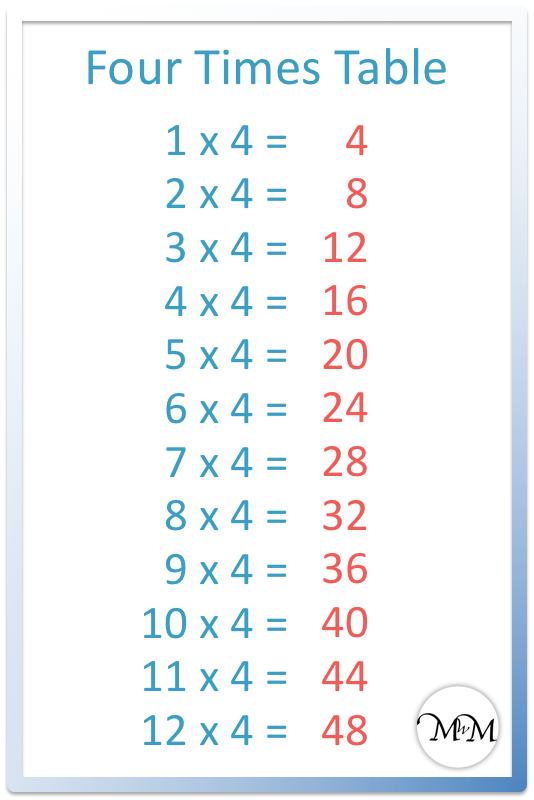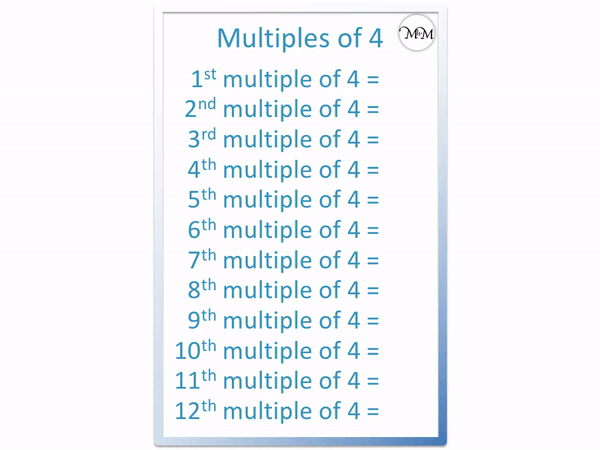#### Multiples of 4 Flashcards

Click on the multiples of 4 flashcards below to memorise the four times table.

×
×

=#### Multiples of 4 Interactive Question Generator

Multiples of 4: Interactive Questions

# Multiples of 4

## What are the Multiples of 4?

Multiples of 4 are the numbers in the four times table such as 4, 8, 12, 16, 20, 24, 28, 32, 36, 40 and so on. Multiples of 4 are always divisible by 4.

Here is a list of all of the multiples of 4 to 100.

4, 8, 12 ,16, 20, 24, 28, 32, 36, 40, 44, 48, 52, 56, 60, 64, 68, 72, 76, 80, 84, 88, 92, 96, 100.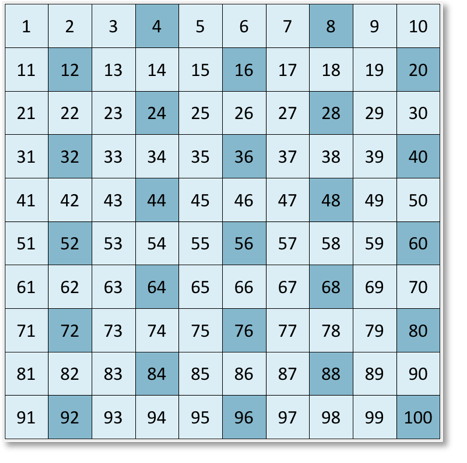Here is a list of all of the multiples of 4 all the way up to 100, shown on a number grid.

We can see that the numbers always appear in certain columns. The multiples of 4 only appear in the even number columns with numbers ending in 0, 2, 4, 6 or 8.However, not all numbers that end in 2, 4, 6, 8 or 0 are multiples of 4. For example 22 is not a multiple of 4 even though it ends in a 2 and 34 is not a multiple of 4 even though it ends in a 4.

List of the multiples of 4 up to 50:

• The 1st multiple of 4 is 4
• The 2nd multiple of 4 is 8
• The 3rd multiple of 4 is 12
• The 4th multiple of 4 is 16
• The 5th multiple of 4 is 20
• The 6th multiple of 4 is 24
• The 7th multiple of 4 is 28
• The 8th multiple of 4 is 32
• The 9th multiple of 4 is 36
• The 10th multiple of 4 is 40
• The 11th multiple of 4 is 44
• The 12th multiple of 4 is 48

## How to Calculate Multiples of 4

To find a multiple of 4, simply multiply any whole number by four. For example, the one hundredth multiple of 4 is 400 because 100 × 4 = 400.For example, to find the 5th multiple of 4, multiply 5 by 4.

5 × 4 = 20

And so, the fifth multiple of four is 20.

The four times table is:

• 1 × 4 = 4
• 2 × 4 = 8
• 3 × 4 = 12
• 4 × 4 = 16
• 5 × 4 = 20
• 6 × 4 = 24
• 7 × 4 = 28
• 8 × 4 = 32
• 9 × 4 = 36
• 10 × 4 = 40
• 11 × 4 = 44
• 12 × 4 = 48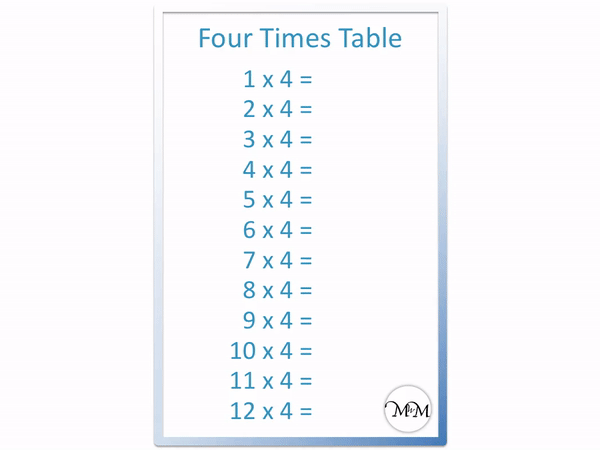## How to Decide if a Number is a Multiple of 4

A number is a multiple of 4 if it can be divided exactly by 4 with no remainder. Halve the number formed by the last two digits of a number. If the result is even, then the original number was a multiple of 4. For example 3044 is a multiple of 4 because 44 ÷ 2 = 22, which is even.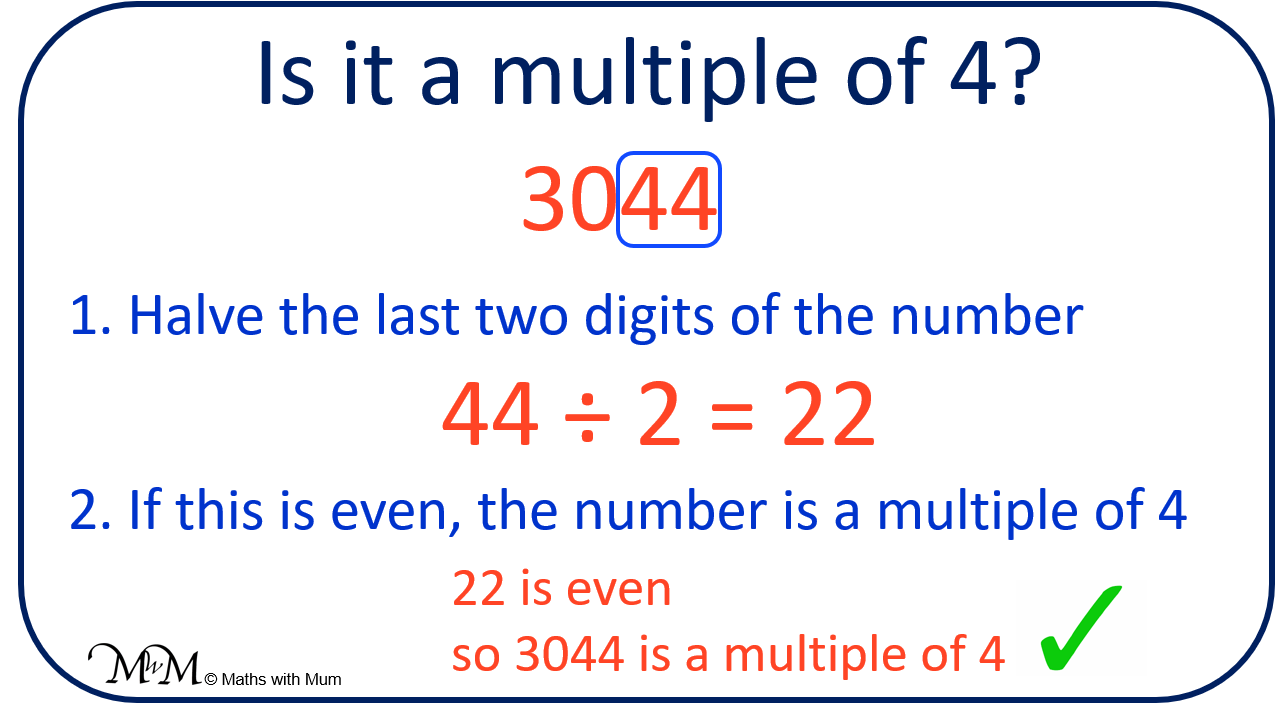This trick works because a number is divisible by 4 if its last 2 digits are divisible by 4.

A number is divisible by 4 if it can be halved and halved again. Therefore if it is halved once and the result is even, we know that it can be halved again. We don’t actually need to halve it again.

To find a multiple of 4, its last two digits must be either 04, 08, 12, 16, 20, 24, 28, 32, 36, 40, 44, 48, 52, 56, 60, 64, 68, 72, 76, 80, 84, 88, 92, 96 or 00. If the number ends in different digits, it is not a multiple of 4.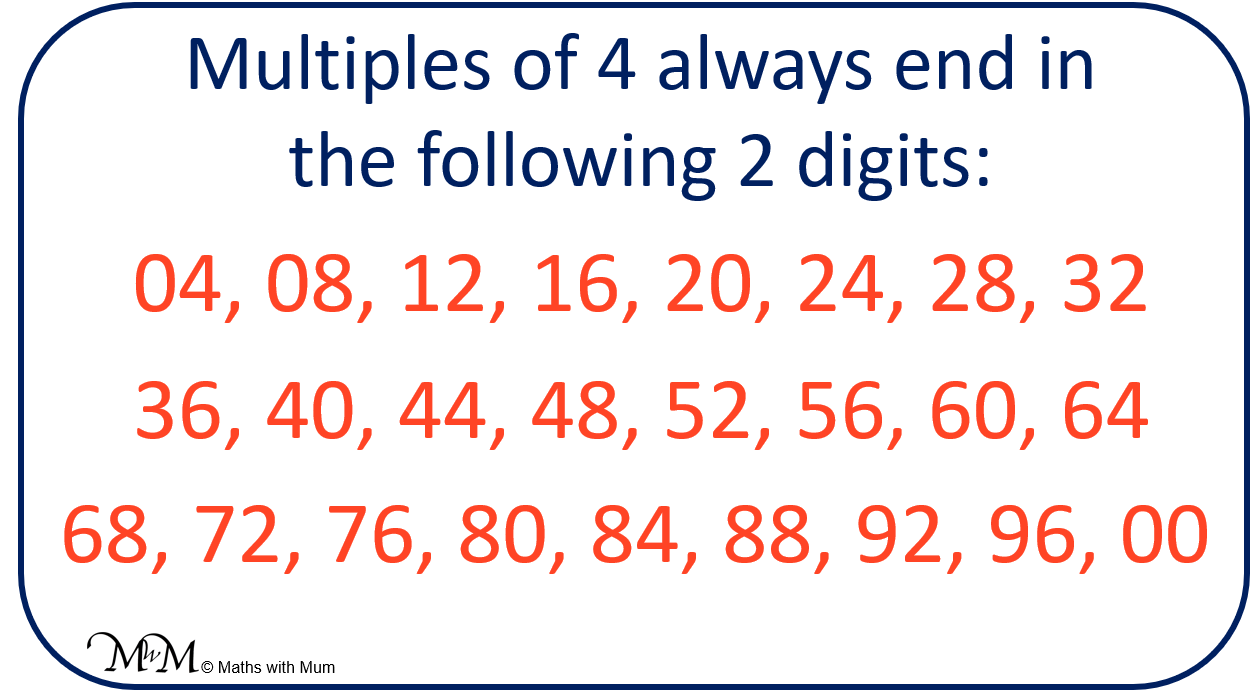E.g. Is 280 a multiple of 4?

Yes because its last two digits are 80. 80 is divisible by 4 and so, 280 is divisible by 4.

E.g. Is 4021 a multiple of 4?

No because its last two digits are 21. 21 is not divisible by 4 and so, 4021 is not divisible by 4.

## Teaching the Multiples of 4

All multiples of 4 end in the same digits, which are 0, 4, 8, 2, 6.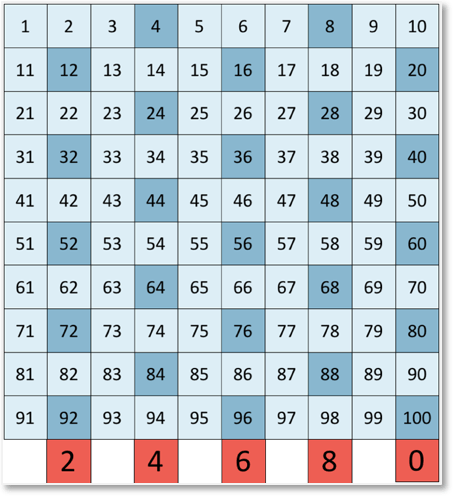This pattern repeats in this order. To get to the next multiple of 4 from the previous multiple of 4, simply add 4.

All multiples of 4 are also multiples of 2. This is because 4 is in the two times table and all multiples of 4 can be divided by 2. All multiples of 4 are even.

When teaching the four times table, we can simply start with the two times table, missing out every other number. If you get stuck, you can count on from the previous number in fours.

The two times table is shown below, with the multiples of 4 shown in bold.

2, 4, 6, 8, 10, 12, 14, 16, 18, 20, 22, 24, 26, 28, 30, 32, 34, 36, 38, 40, 42, 44, 46, 48.We can also teach this by thinking of it as doubling every number in the two times table.Now try our lesson on Finding the Lowest Common Multiple where we learn about the lowest common multiple.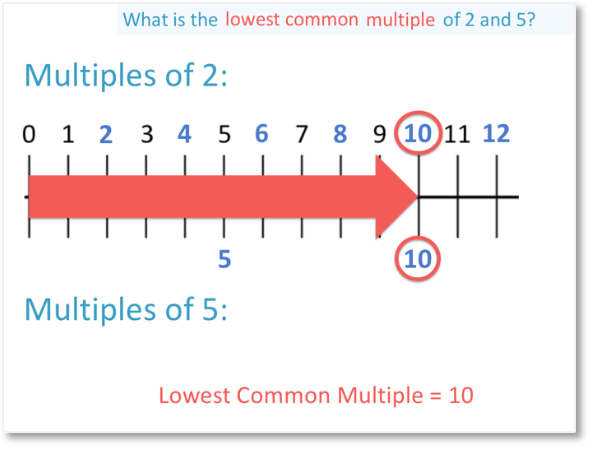error: Content is protected !!